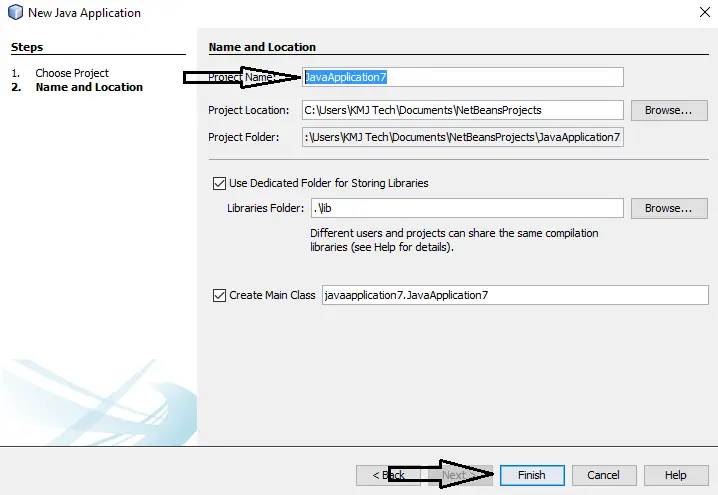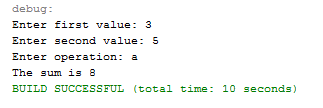# How to Create Basic Calculator using Java

0
322

This tutorial will teach you how to create a basic calculator using if statement in Java. This calculator can calculate using the four operations of mathematics.

I am using “if Statement” to identify what operation should use in calculating two numbers. And also a “Boolean” condition if statement, it means that only two results of the condition should display if it is the condition is true or false. I am also using a “Scanner” Function so that the user can input the values when the program is running. Please follow all the steps to complete this tutorial.

### Basic Calculator Using If Statements Steps

1. Create a new project.2. Go to your source view and insert the code below above your class.

[java]import java.util.Scanner;[/java]

3. Inside your main method, declare five variables. Your code should look like below.

[java]Scanner input = new Scanner(System.in);
int num1=0,num2=0,total=0;
String op=””;[/java]

Input – scanner variable

Num1 – handle the first value

Num2 – handle the second value

Total – display the result

Op – handle the input operation

4. Insert the statement below after step 3. This statement will ask the user input of first value, the second value, and operation.

[java]System.out.print(“Enter first value: “);
num1 = input.nextInt();
System.out.print(“Enter second value: “);
num2 = input.nextInt();
System.out.print(“Enter operation: “);
op = input.next();[/java]

5. Insert the statement below after step 4. The following statement will execute the algorithm and display the result.

[java]if (op.equals(“a”)){
total = num1 + num2;
System.out.println(“The sum is ” + total);
}else if(op.equals(“s”)){
total = num1 – num2;
System.out.println(“The difference is ” + total);
}else if(op.equals(“m”)){
total = num1 * num2;
System.out.println(“The product is ” + total);
}else if(op.equals(“d”)){
total = num1 / num2;
System.out.println(“The quotient is ” + total);
}else{
System.out.println(“Wrong operation!”);
}[/java]

6. Run your program and the result should look like the image below.7. Complete Source Code.

[java]import java.util.Scanner;
public class BasicCalculator {
public static void main(String args[]){
//declaring variables
Scanner input = new Scanner(System.in);
int num1=0,num2=0,total=0;
String op=””;
//initializing the variables
System.out.print(“Enter first value: “);
num1 = input.nextInt();
System.out.print(“Enter second value: “);
num2 = input.nextInt();
System.out.print(“Enter operation: “);
op = input.next();
//runing the algo and display result
if (op.equals(“a”)){
total = num1 + num2;
System.out.println(“The sum is ” + total);
}else if(op.equals(“s”)){
total = num1 – num2;
System.out.println(“The difference is ” + total);
}else if(op.equals(“m”)){
total = num1 * num2;
System.out.println(“The product is ” + total);
}else if(op.equals(“d”)){
total = num1 / num2;
System.out.println(“The quotient is ” + total);
}else{
System.out.println(“Wrong operation!”);
}
}
}[/java]

REMEMBER:
Operation “a” for addition, “s” for subtraction, “m” for multiplication, “d” for division. If you input operation in another format, the program will display message “Wrong operation”.

## If you have any comments or suggestions about on How to Create Basic Calculator using Java, feel free to leave your comment below, use the contact page of this website or use my contact information.

Email: [email protected]  |  Cell. No.: 09468362375